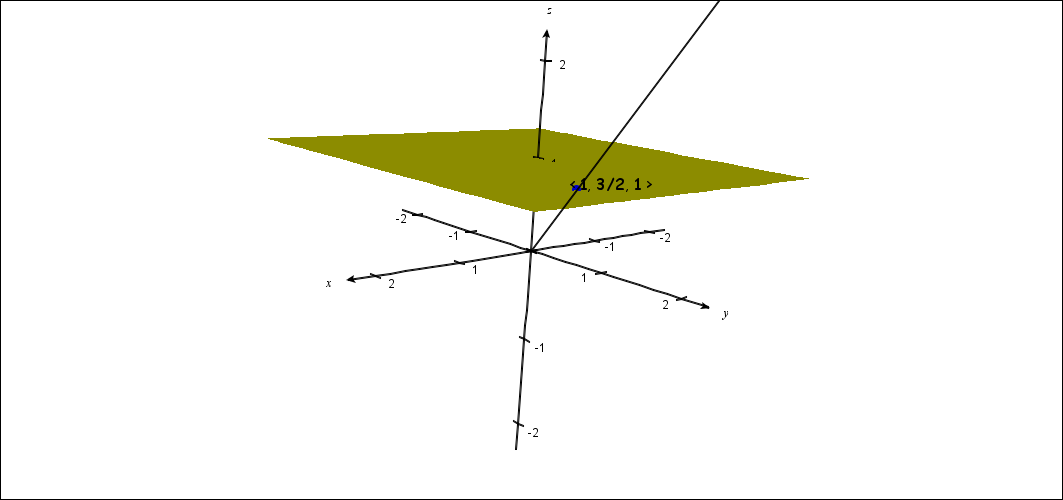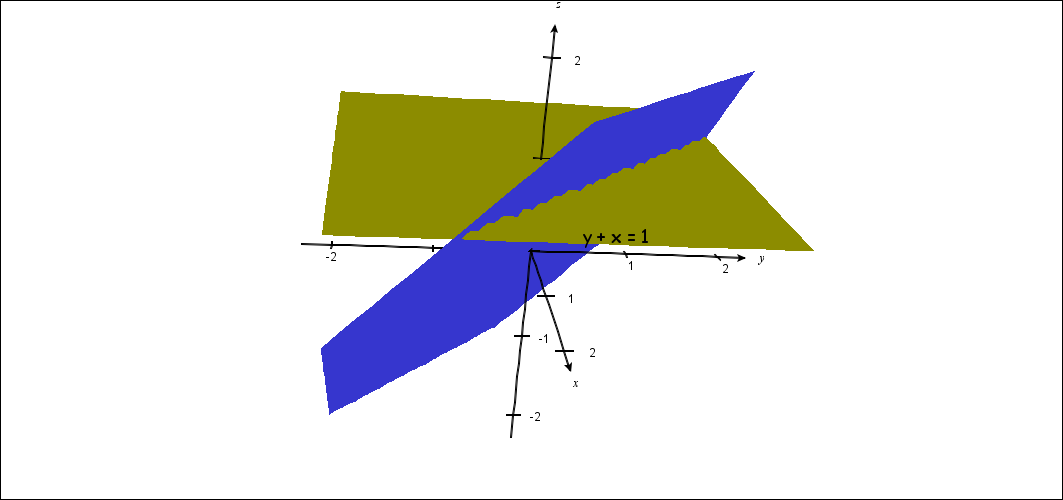# Objects in  $RP^2$

Definition: A line in $RP^2$ : $L=\{$ <  $x,y,z$ > $\in RP^2 : Ax + By + Cz = 0, (A,B,C) \ne (0,0,0).\} = [A,B,C]$
A line in $RP^2$  "=" A plane through $(0,0,0)$ in $R^3 - \{(0,0,0)\}$.

The ideal line in $RP^2$ : $RP^2 - R^2 =$ {< $x,y,0$ >  $: x,y \in R\} = [0,0,1]$.

The ideal line in $RP^2$  "=" The $XY$ plane in $R^3 - \{(0,0,0)\}$.

Summary: A point in $RP^2$ corresponds to a line in $R^3$ that passes through $(0,0,0)$.
A line in $RP^2$ corresponds to a plane in $R^3$ that passes through $(0,0,0)$.

 A Point in $RP^2$ : a Line in $R^3$A line in $RP^2$ : a Plane in $R^3$